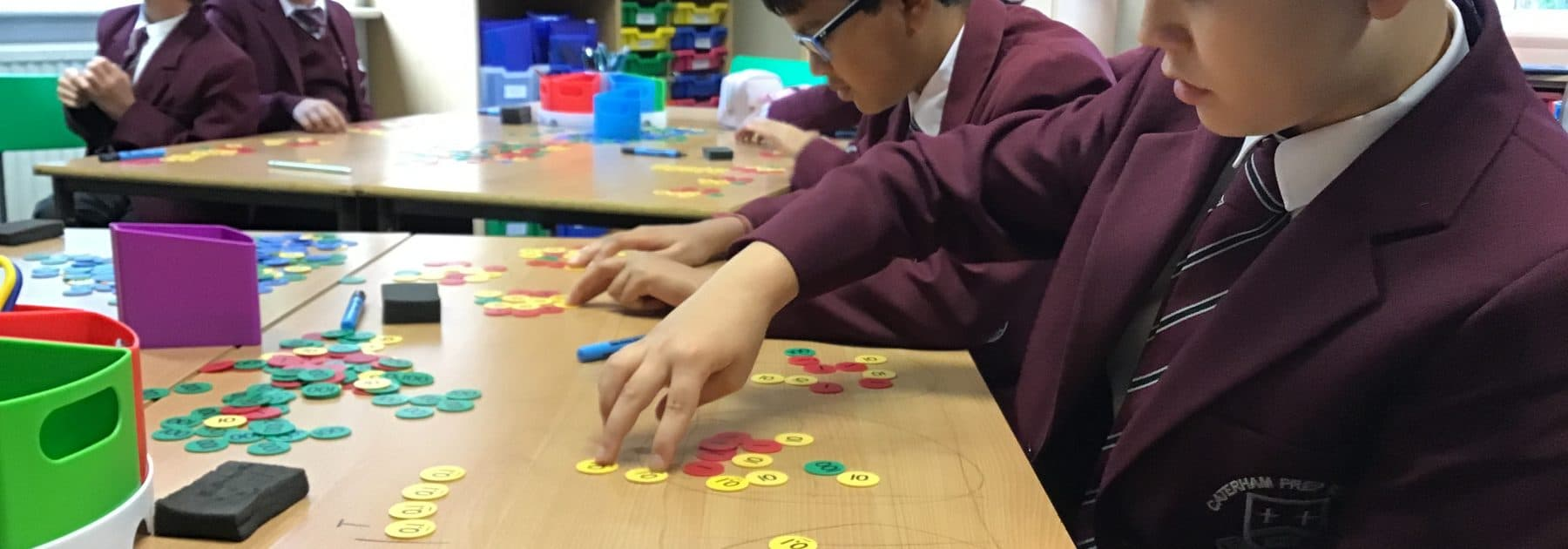# A Place (Value) for Everything

True mathematicians aren’t just able to apply a rule, but understand how Maths works so that they can use it effectively. In their Maths lessons, children in Years 3-6 have been learning and talking about what the core operations (addition, subtraction, multiplication and division) actually are and how we can use them to solve problems practically as well as with pen and paper.

Decimals don’t have to be daunting! Year 6 have been looking at place value in division. Rather than simply ‘carrying the one’, they have explored what it actually looks like when we divide a large number into smaller pieces or exchange a 1 into 10 tenths, a tenth into 10 hundredth and further on into increasingly smaller decimals. Gaining a true understanding of how numbers and operation work is the difference between using a rule and understanding it.